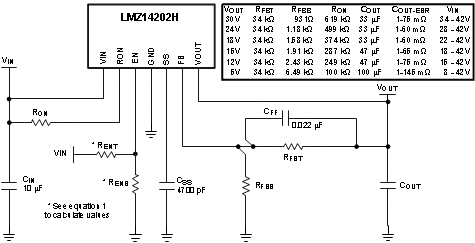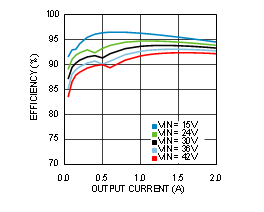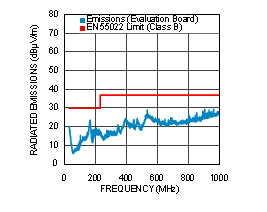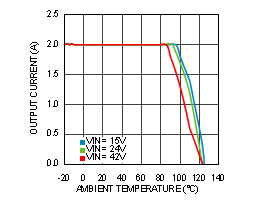SNVS691H January   2011  – October 2015

PRODUCTION DATA.

1. Features
2. Applications
3. Description
4. Revision History
5. Pin Configuration and Functions
6. Specifications
7. Detailed Description
1. 7.1 Overview
2. 7.2 Functional Block Diagram
3. 7.3 Feature Description
4. 7.4 Device Functional Modes
8. Application and Implementation
1. 8.1 Application Information
2. 8.2 Typical Application
1. 8.2.1 Design Requirements
2. 8.2.2 Detailed Design Procedure
1. 8.2.2.1 Design Steps for the LMZ14202H Application
3. 8.2.3 Application Curves
9. Power Supply Recommendations
10. 10Layout
11. 11Device and Documentation Support
12. 12Mechanical, Packaging, and Orderable Information

• NDW|7

## 8 Application and Implementation

NOTE

Information in the following applications sections is not part of the TI component specification, and TI does not warrant its accuracy or completeness. TI’s customers are responsible for determining suitability of components for their purposes. Customers should validate and test their design implementation to confirm system functionality.

### 8.1 Application Information

The LMZ14202H is a step-down DC-to-DC power module. It is typically used to convert a higher DC voltage to a lower DC voltage with a maximum output current of 2 A. The following design procedure can be used to select components for the LMZ14202H. Alternately, the WEBENCH software may be used to generate complete designs.

When generating a design, the WEBENCH software uses iterative design procedure and accesses comprehensive databases of components. For more details, go to www.ti.com.

### 8.2 Typical ApplicationFigure 46. Typical Application Schematic

#### 8.2.1 Design Requirements

For this example the following application parameters exist.

• VIN Range = Up to 42 V
• VOUT = 5 V to 30 V
• IOUT = 2 A

#### 8.2.2.1 Design Steps for the LMZ14202H Application

The LMZ14202H is fully supported by WEBENCH which offers the following:

• Component selection
• Electrical simulation
• Thermal simulation
• Build-it prototype board for a reduction in design time

The following list of steps can be used to manually design the LMZ14202H application.

1. Select minimum operating VIN with enable divider resistors.
2. Program VO with divider resistor selection.
3. Program turnon time with soft-start capacitor selection.
4. Select CO.
5. Select CIN.
6. Set operating frequency with RON.
7. Determine module dissipation.
8. Lay out PCB for required thermal performance.

#### 8.2.2.1.1 Enable Divider, RENT and RENB Selection

The enable input provides a precise 1.18-V reference threshold to allow direct logic drive or connection to a voltage divider from a higher enable voltage such as VIN. The enable input also incorporates 90 mV (typical) of hysteresis resulting in a falling threshold of 1.09V. The maximum recommended voltage into the EN pin is 6.5 V. For applications where the midpoint of the enable divider exceeds 6.5 V, a small Zener diode can be added to limit this voltage.

The function of the RENT and RENB divider shown in the Functional Block Diagram is to allow the designer to choose an input voltage below which the circuit will be disabled. This implements the feature of programmable under voltage lockout. This is often used in battery-powered systems to prevent deep discharge of the system battery. It is also useful in system designs for sequencing of output rails or to prevent early turnon of the supply as the main input voltage rail rises at power up. Applying the enable divider to the main input rail is often done in the case of higher input voltage systems such as 24-V AC/DC systems where a lower boundary of operation should be established. In the case of sequencing supplies, the divider is connected to a rail that becomes active earlier in the power-up cycle than the LMZ14202H output rail. The two resistors should be chosen based on the following ratio:

Equation 1. RENT / RENB = (VIN-ENABLE/ 1.18 V) – 1

The EN pin is internally pulled up to VIN and can be left floating for always-on operation. However, it is good practice to use the enable divider and turn on the regulator when VIN is close to reaching its nominal value. This will ensure smooth start-up and will prevent overloading the input supply.

#### 8.2.2.1.2 Output Voltage Selection

Output voltage is determined by a divider of two resistors connected between VO and ground. The midpoint of the divider is connected to the FB input. The voltage at FB is compared to a 0.8-V internal reference. In normal operation an ON-time cycle is initiated when the voltage on the FB pin falls below 0.8 V. The high-side MOSFET ON-time cycle causes the output voltage to rise and the voltage at the FB to exceed 0.8 V. As long as the voltage at FB is above 0.8 V, ON-time cycles will not occur.

The regulated output voltage determined by the external divider resistors RFBT and RFBB is:

Equation 2. VO = 0.8 V × (1 + RFBT / RFBB)

Rearranging terms; the ratio of the feedback resistors for a desired output voltage is:

Equation 3. RFBT / RFBB = (VO / 0.8 V) - 1

These resistors should be chosen from values in the range of 1 kΩ to 50 kΩ.

A feed-forward capacitor is placed in parallel with RFBT to improve load step transient response. Its value is usually determined experimentally by load stepping between DCM and CCM conduction modes and adjusting for best transient response and minimum output ripple.

A table of values for RFBT , RFBB , and RON is included in the simplified applications schematic.

#### 8.2.2.1.3 Soft-Start Capacitor, CSS, Selection

Programmable soft-start permits the regulator to slowly ramp to its steady state operating point after being enabled, thereby reducing current inrush from the input supply and slowing the output voltage rise-time to prevent overshoot.

Upon turnon, after all UVLO conditions have been passed, an internal 8-uA current source begins charging the external soft-start capacitor. The soft-start time duration to reach steady state operation is given by the formula:

Equation 4. tSS = VREF × CSS / Iss = 0.8 V × CSS / 8uA

This equation can be rearranged as follows:

Equation 5. CSS = tSS × 8 μA / 0.8 V

Use of a 4700-pF capacitor results in 0.5ms soft-start duration. This is a recommended value. Note that high values of CSS capacitance will cause more output voltage droop when a load transient goes across the DCM-CCM boundary. Use Equation 22 below to find the DCM-CCM boundary load current for the specific operating condition. If a fast load transient response is desired for steps between DCM and CCM mode the soft-start capacitor value should be less than 0.018µF.

As the soft-start input exceeds 0.8 V the output of the power stage will be in regulation. Note that the following conditions will reset the soft-start capacitor by discharging the SS input to ground with an internal 200 μA current sink:

• The enable input being “pulled low”
• Thermal shutdown condition
• Overcurrent fault
• Internal VINUVLO

#### 8.2.2.1.4 Output Capacitor, CO, Selection

None of the required output capacitance is contained within the module. At a minimum, the output capacitor must meet the worst-case RMS current rating of 0.5 x ILR P-P, as calculated in Equation 23. Beyond that, additional capacitance will reduce output ripple so long as the ESR is low enough to permit it. A minimum value of 10 μF is generally required. Experimentation will be required if attempting to operate with a minimum value. Low ESR capacitors, such as ceramic and polymer electrolytic capacitors are recommended.

#### 8.2.2.1.4.1 Capacitance

Equation 6 provides a good first pass approximation of CO for load transient requirements:

Equation 6. CO≥ISTEP x VFB x L x VIN/ (4 x VO x (VIN — VO) x VOUT-TRAN)

As an example, for 2-A load step, VIN = 24 V, VOUT = 12 V, VOUT-TRAN = 50 mV:

Equation 7. CO≥ 2 A x 0.8 V x 15 μH x 24 V / (4 x 12 V x ( 24 V — 12 V) x 50 mV)
Equation 8. CO≥ 20 μF

#### 8.2.2.1.4.2 ESR

The ESR of the output capacitor affects the output voltage ripple. High ESR will result in larger VOUT peak-to-peak ripple voltage. Furthermore, high output voltage ripple caused by excessive ESR can trigger the overvoltage protection monitored at the FB pin. The ESR should be chosen to satisfy the maximum desired VOUT peak-to-peak ripple voltage and to avoid overvoltage protection during normal operation. The following equations can be used:

Equation 9. ESRMAX-RIPPLE ≤ VOUT-RIPPLE / ILR P-P

where

Equation 10. ESRMAX-OVP < (VFB-OVP - VFB) / (ILR P-P x AFB )

where

• AFB is the gain of the feedback network from VOUT to VFB at the switching frequency.

As worst-case, assume the gain of AFB with the CFF capacitor at the switching frequency is 1.

The selected capacitor should have sufficient voltage and RMS current rating. The RMS current through the output capacitor is:

Equation 11. I(COUT(RMS)) = ILR P-P / √12

#### 8.2.2.1.5 Input Capacitor, CIN, Selection

The LMZ14202H module contains an internal 0.47 µF input ceramic capacitor. Additional input capacitance is required external to the module to handle the input ripple current of the application. This input capacitance should be as close as possible to the module. Input capacitor selection is generally directed to satisfy the input ripple current requirements rather than by capacitance value. Worst-case input ripple current rating is dictated by Equation 12:

Equation 12. I(CIN(RMS)) ≊ 1 / 2 x IO x √ (D / 1-D)

where

• D ≊ VO / VIN

(As a point of reference, the worst-case ripple current will occur when the module is presented with full load current and when VIN = 2 x VO).

Recommended minimum input capacitance is 10-uF X7R ceramic with a voltage rating at least 25% higher than the maximum applied input voltage for the application. It is also recommended that attention be paid to the voltage and temperature deratings of the capacitor selected. It should be noted that ripple current rating of ceramic capacitors may be missing from the capacitor data sheet and you may have to contact the capacitor manufacturer for this rating.

If the system design requires a certain maximum value of input ripple voltage ΔVIN to be maintained then Equation 13 may be used.

Equation 13. CIN ≥ IO x D x (1–D) / fSW-CCM x ΔVIN

If ΔVIN is 1% of VIN for a 24V input to 12V output application this equals 240 mV and fSW = 400 kHz.

Equation 14. CIN≥ 2 A x 12 V/24 V x (1– 12 V/24 V) / (400000 x 0.240 V)
Equation 15. CIN≥ 5.2 μF

Additional bulk capacitance with higher ESR may be required to damp any resonant effects of the input capacitance and parasitic inductance of the incoming supply lines.

#### 8.2.2.1.6 ON-Time, RON, Resistor Selection

Many designs will begin with a desired switching frequency in mind. As seen in the Typical Characteristics section, the best efficiency is achieved in the 300kHz-400kHz switching frequency range. Equation 16 can be used to calculate the RON value.

Equation 16. fSW(CCM) ≊ VO / (1.3 x 10-10 x RON)

This can be rearranged as

Equation 17. RON ≊ VO / (1.3 x 10 -10 x fSW(CCM)

The selection of RON and fSW(CCM) must be confined by limitations in the ON-time and OFF-time for the COT Control Circuit Overview section.

The ON-time of the LMZ14202H timer is determined by the resistor RON and the input voltage VIN. It is calculated as follows:

Equation 18. tON = (1.3 x 10-10 x RON) / VIN

The inverse relationship of tON and VIN gives a nearly constant switching frequency as VIN is varied. RON should be selected such that the ON-time at maximum VIN is greater than 150 ns. The ON-timer has a limiter to ensure a minimum of 150 ns for tON. This limits the maximum operating frequency, which is governed by Equation 19:

Equation 19. fSW(MAX) = VO / (VIN(MAX) x 150 nsec)

This equation can be used to select RON if a certain operating frequency is desired so long as the minimum ON-time of 150 ns is observed. The limit for RON can be calculated as follows:

Equation 20. RON ≥ VIN(MAX) x 150 nsec / (1.3 x 10 -10)

If RON calculated in Equation 17 is less than the minimum value determined in Equation 20 a lower frequency should be selected. Alternatively, VIN(MAX) can also be limited in order to keep the frequency unchanged.

Additionally, the minimum OFF-time of 260 ns (typical) limits the maximum duty ratio. Larger RON (lower FSW) should be selected in any application requiring large duty ratio.

#### 8.2.2.1.6.1 Discontinuous Conduction and Continuous Conduction Modes Selection

Operating frequency in DCM can be calculated as follows:

Equation 21. fSW(DCM)≊VO x (VIN-1) x 15 μH x 1.18 x 1020 x IO / (VIN–VO) x RON2

In CCM, current flows through the inductor through the entire switching cycle and never falls to zero during the OFF-time. The switching frequency remains relatively constant with load current and line voltage variations. The CCM operating frequency can be calculated using Equation 16 above.

The approximate formula for determining the DCM/CCM boundary is as follows:

Equation 22. IDCB≊VOx (VIN–VO) / ( 2 x 15μH x fSW(CCM) x VIN)

The inductor internal to the module is 15 μH. This value was chosen as a good balance between low and high input voltage applications. The main parameter affected by the inductor is the amplitude of the inductor ripple current (ILR). ILR can be calculated with:

Equation 23. ILR P-P=VO x (VIN- VO) / (15 µH x fSW x VIN)

where

• VIN is the maximum input voltage and fSW is determined from Equation 16.

If the output current IO is determined by assuming that IO = IL, the higher and lower peak of ILR can be determined. Be aware that the lower peak of ILR must be positive if CCM operation is required.

#### 8.2.3 Application CurvesFigure 47. Efficiency VOUT = 12 VFigure 49. Radiated Emissions (EN 55022 Class B)Figure 48. Thermal Derating VOUT = 12 V, RθJA = 16°C/W